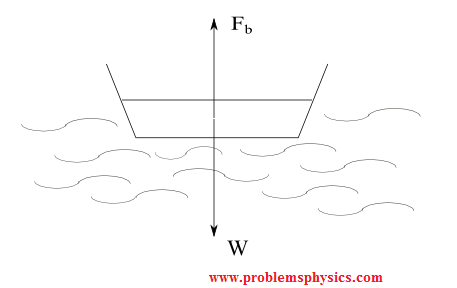# Forces in Physics

## What are Forces in Physics?

A force describes, quantitatively, the interaction between two objects. A force is a vector quantity: its has a magnitude and a direction. You need to exert a force to pull a box on the floor. You use force to push a table. The weight of an object is the force of attraction that the earth exerts on this object. This interaction may be at a distance or through contact. Forces are used to push or pull stationary objects, to stop moving objects, to change direction of moving objects.
Forces always exist in pairs: a force of action and a force of reaction (third law of Newton). Action and reaction forces acts on different objects and have equal magnitudes.
A force is represented mathematically by a vector because both magnitude and direction are important to describe the force.
The SI unit of a force is the Newton. 1 Newton = 1 Kg � 1 m/s
2
Therefore, the newton (N) is the magnitude of the force applied to an object of a mass of 1 Kg in order to acquire an acceleration of 1 m/s
2.

## Classification of Forces

A force can be put in one of the two classes: Forces with contact and forces at a distance.

### A) Examples of forces with contact

1) The force exerted by person to push a trolley
2) The force (tension) exerted by a string on a suspended object
3) The force exerted by the ground on a table in a room (normal force)

### B) Examples of Forces at a distance (also called field forces)

1) The force exerted by a planet (Earth for example) on any object with a mass.
2) The force exerted by an magnet on another magnet.
3) The electric force exerted by an electric charge on another electric charge.

## Types of forces acting on objects

• ### Applied External Force

Any force applied to an object externally by another object, human or animal. A person that is pushing a box, is applying an external force on the box.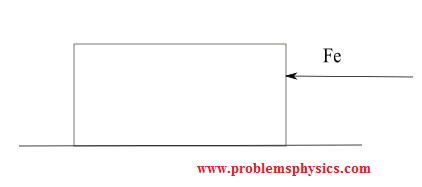• ### Gravitational Force

It is the force exerted by the earth on any object with a mass. This force is due to the gravitational field of the earth. It is also called the weight of an object.• ### Electric Force on a Charge

It is the force exerted on a charge that is located in an electric field. Two forces of equal magnitudes and opposite direction act on two charges of different signs in the figure below.• ### Magnetic Force

It is the force exerted on a magnet that is located in a magnetic field. Two forces of equal magnitude and opposite direction acts on each of the magnets in the figure below.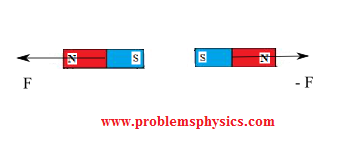• ### Normal Force

The normal force is the force exerted by an object onto another object when in contact. Example of a box on a table shown in the figure below. The table exerts a normal force N on the box.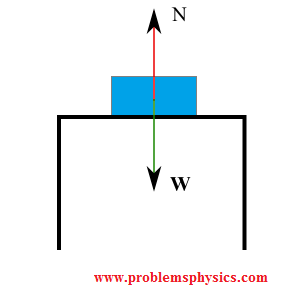• ### Force of Friction

A force of friction is exerted by one object on another as one them moves or tries to move relative to the other. In general, a force of friction opposes the motion of objects. There are two types of force of friction: static and kinetic. The static force of friction is exerted on an object that tries to move. A kinetic force of friction is exerted on an object that is in motion.• ### Tension in strings, ropes, ...

Tension is a force transmitted by means of a flexible object such as a string, rope, ... between two points of a system. The main characteristic of a tension is that it has the same magnitude at every point of the string, neglecting the mass of the string. It may or may not have the same direction at different points of the string.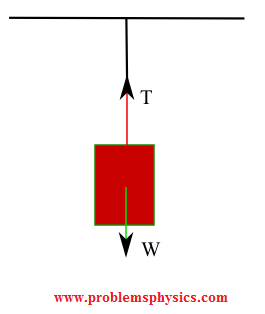• ### Force of reaction

The reaction to air rushing down out of a balloon is the balloon moving upward. The forces of action and reaction have equal magnitude.• ### Air Resistance Force

Force due to air that tends to oppose any movement of an object in air. It is another type of frictional force. An example below of a car moving from right to left and the force due to air resistance pushing the car to the right.• ### Upthrust (or buoyancy) Force in a Liquid or gas

Any object immersed in a fluid (liquid or gas) is thrust upward by a force exerted by the fluid that opposes its weight. This force, called upthrust force or buoyancy force explains why boats, for example, stays afloat on water.